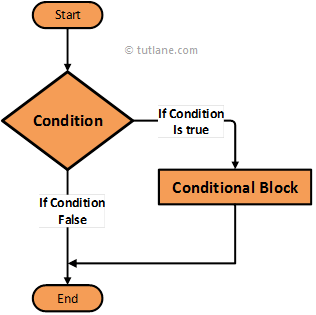# Swift If Statement

In swift, if statement or condition is useful to execute a set of statements if defined condition is TRUE.

Whenever we have specific requirements like execute a set of block statements only if a given condition is TRUE then we can use swift if statement.

Now we will see the functionality of if statement in swift programming language using algorithm diagram.

## Swift If Statement Flow Diagram

Following is the swift if statement flow diagram to represent how the functionality of if statement will work in a swift programming language.If you observe above swift if condition flow diagram first it will evaluates the given condition is TRUE or FALSE. If the given condition is satisfied (TRUE) then it will execute the code block within if statement. In case the given condition is FALSE then if condition block will skip the execution of given code block and reach the end of statement.

## Syntax of Swift If Statement

Following is the syntax of defining if statement in swift programming language.

if condition

{

//Expressions will execute only when condition true

}

If you observe above if statement syntax if defined "condition" TRUE then only the statements inside of if statement will execute.

Now we will see how to use if statement with examples in swift programming language.

## Swift If Statement Example

Following is the simple example using if statement in swift to check the condition before execute the expressions within the condition.

var num1: Int = 40

if num1 > 20 {

print("Number Greater than 20")

}

If you observe above example we are checking the condition whether the value of “num1” greater 20 or not.

When we execute the above code in swift playground we will get a result like as shown below.

Number Greater than 20

This is how we can use swift if statement to execute defined expressions based on our requirements.